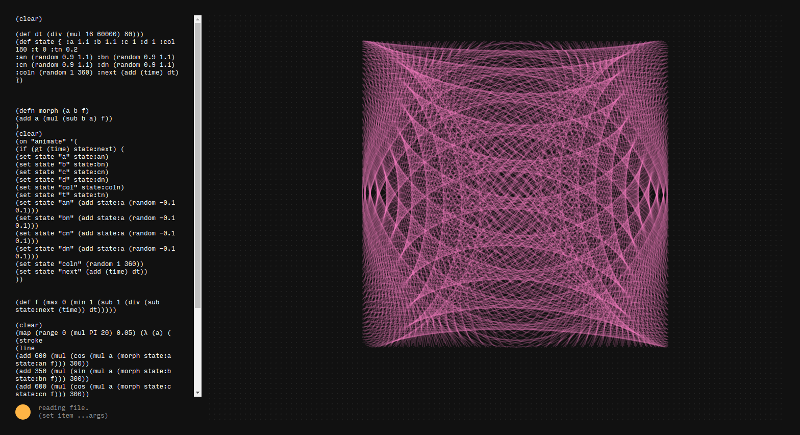## Ronin experiment 21 - morphing lissajous states

this visualizer written in ronin morphes between states of a lissajous figure in sync with the music.

I transition between the states by interpolating the state variables linear over a time periode that is in sync with 4 bars of the music.here is the code i used for this visualizer (def dt (div (mul 16 60000) 80))) (def state { :a 1.1 :b 1.1 :c 1 :d 1 :col 180 :t 0 :tn 0.2 :an (random 0.9 1.1) :bn (random 0.9 1.1) :cn (random 0.9 1.1) :dn (random 0.9 1.1) :coln (random 1 360) :next (add (time) dt) })

``````(defn morph (a b f)
(add a (mul (sub b a) f))
)
(clear)
(on "animate" '(
(if (gt (time) state:next) (
(set state "a" state:an)
(set state "b" state:bn)
(set state "c" state:cn)
(set state "d" state:dn)
(set state "col" state:coln)
(set state "t" state:tn)
(set state "an" (add state:a (random -0.1 0.1)))
(set state "bn" (add state:a (random -0.1 0.1)))
(set state "cn" (add state:a (random -0.1 0.1)))
(set state "dn" (add state:a (random -0.1 0.1)))
(set state "coln" (random 1 360))
(set state "next" (add (time) dt))
))

(def f (max 0 (min 1 (sub 1 (div (sub state:next (time)) dt)))))

(clear)
(map (range 0 (mul PI 20) 0.05) (λ (a) (
(stroke
(line
(add 600 (mul (cos (mul a (morph state:a state:an f))) 300))
(add 350 (mul (sin (mul a (morph state:b state:bn f))) 300))
(add 600 (mul (cos (mul a (morph state:c state:cn f))) 300))
(add 350 (mul (sin (mul a (morph state:d state:dn f))) 300)) )

(hsl (morph state:col state:coln f) 100 75 (morph state:t state:tn f)
1)

)))
))

(on "key-down" '(
(set state "t" 0.2 )
(set state "tn" 0)
))
``````